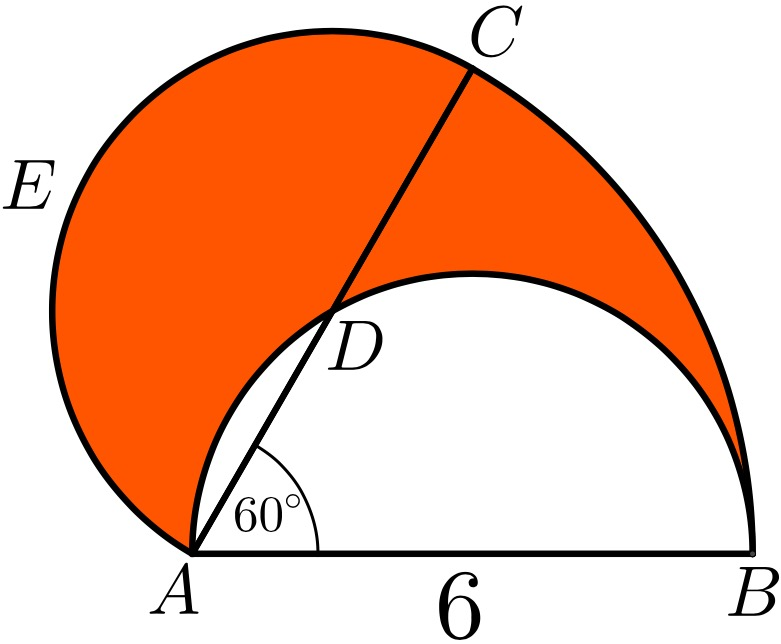# Constructing A Crescent MoonSemicircle $ADB$ with diameter 6 is rotated $60^\circ$ anticlockwise at the point $A$ to form another semicircle $AEC$ with diameter $AC$. If the area of the shaded region can be expressed as $k\pi$, find $k$.

×